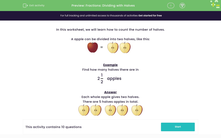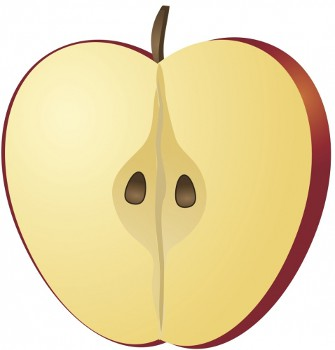# Practise Finding the Number of Halves in Mixed Numbers

In this worksheet, students will evaluate the number of halves in a mixed number.Key stage:  KS 2

Curriculum topic:   Number: Multiplication and Division

Curriculum subtopic:   Solve Multiplication/Division Problems with Fractions

Difficulty level:#### Worksheet Overview

In this worksheet, we will learn how to count the number of halves.

An apple can be divided into two halves, like this:=Example

Find how many halves there are in

2

 1 2

apples

Each whole apple gives two halves.

There are 5 half apples in total.The easiest way to work this out is to multiply the big whole number (2 in this case) by the denominator (the number at the bottom of the fraction - in this case 2)

2 x 2 = 4

Then add the numerator (the number at the top of the fraction - in this case 1)

4 + 1 = 5

Here's another one to try:

How many halves are there in 4 ½ ?

Multiply the whole number (4 ) by the denominator (2): 4 x 2 = 8

Add the numerator (1): 8 + 1 = 9

So there are 9 halves.

Let's try some questions now.

### What is EdPlace?

We're your National Curriculum aligned online education content provider helping each child succeed in English, maths and science from year 1 to GCSE. With an EdPlace account you’ll be able to track and measure progress, helping each child achieve their best. We build confidence and attainment by personalising each child’s learning at a level that suits them.

Get started